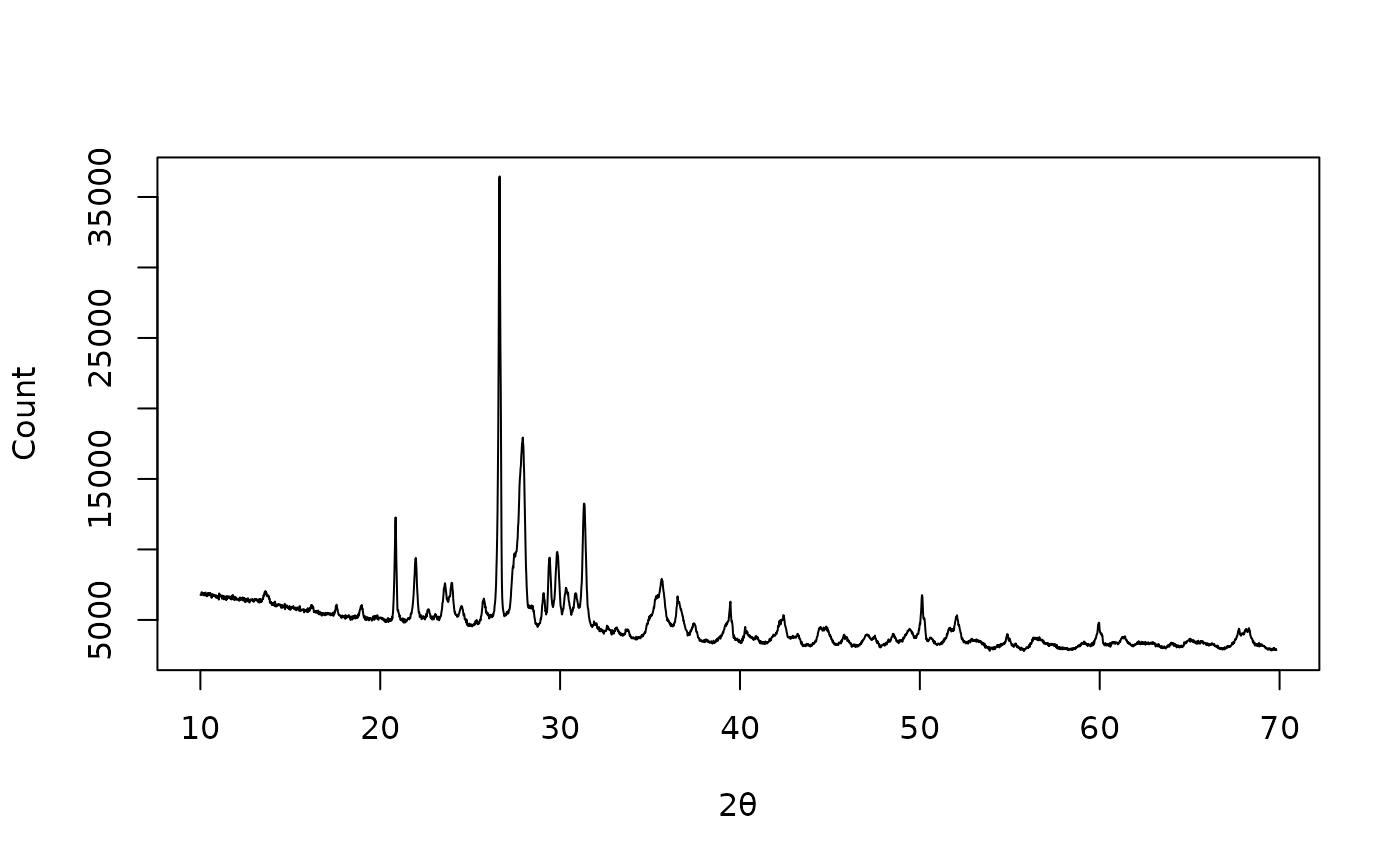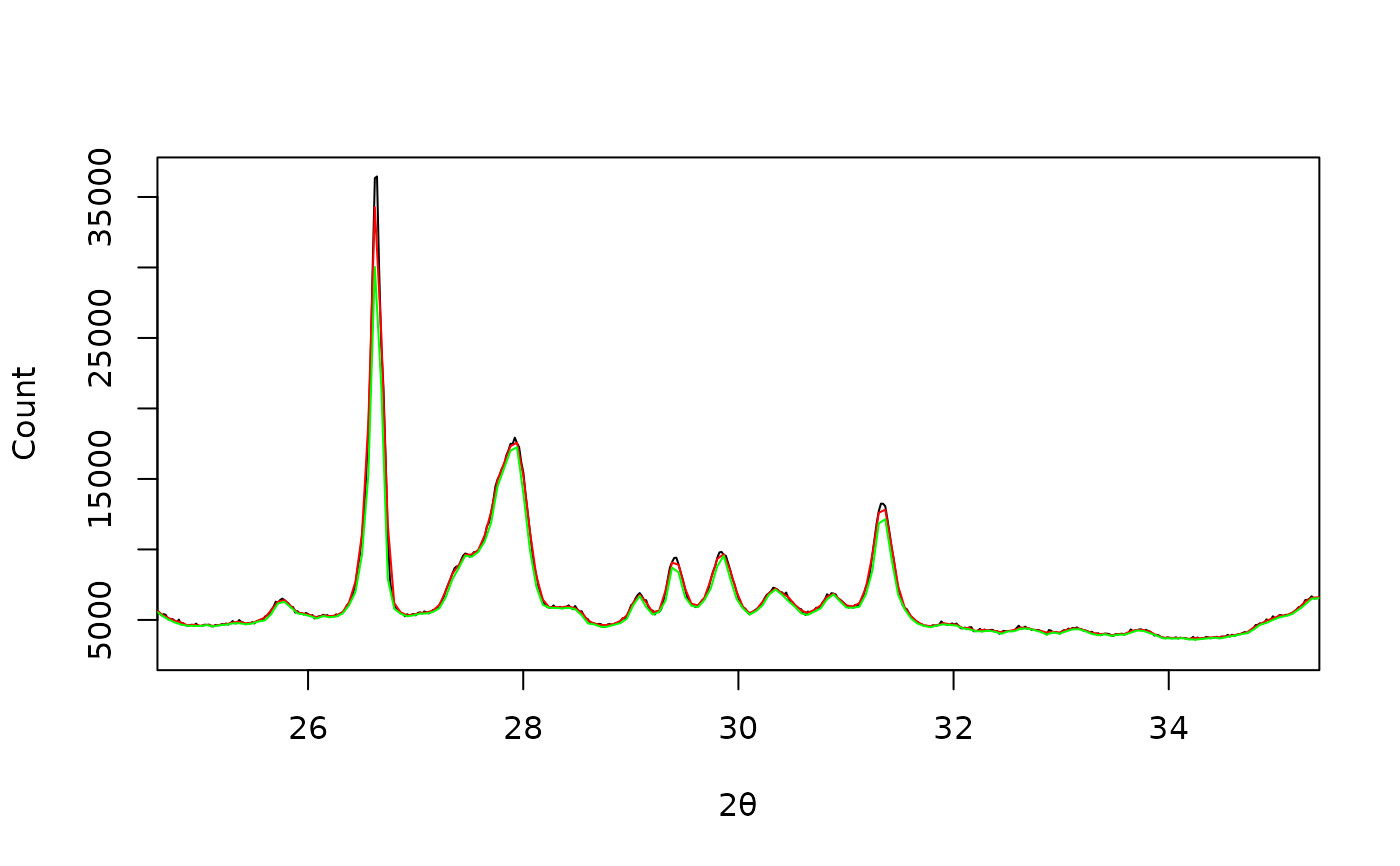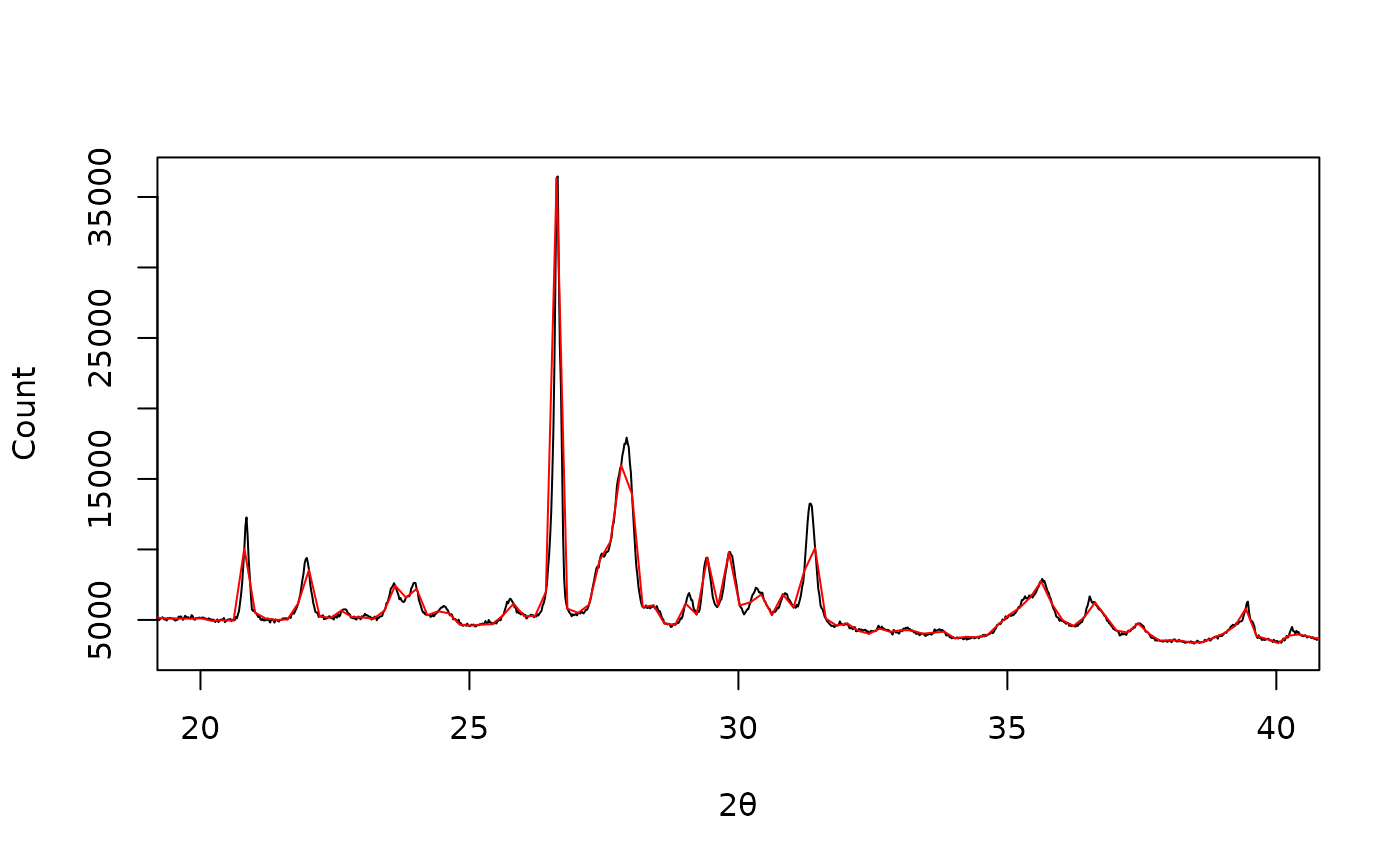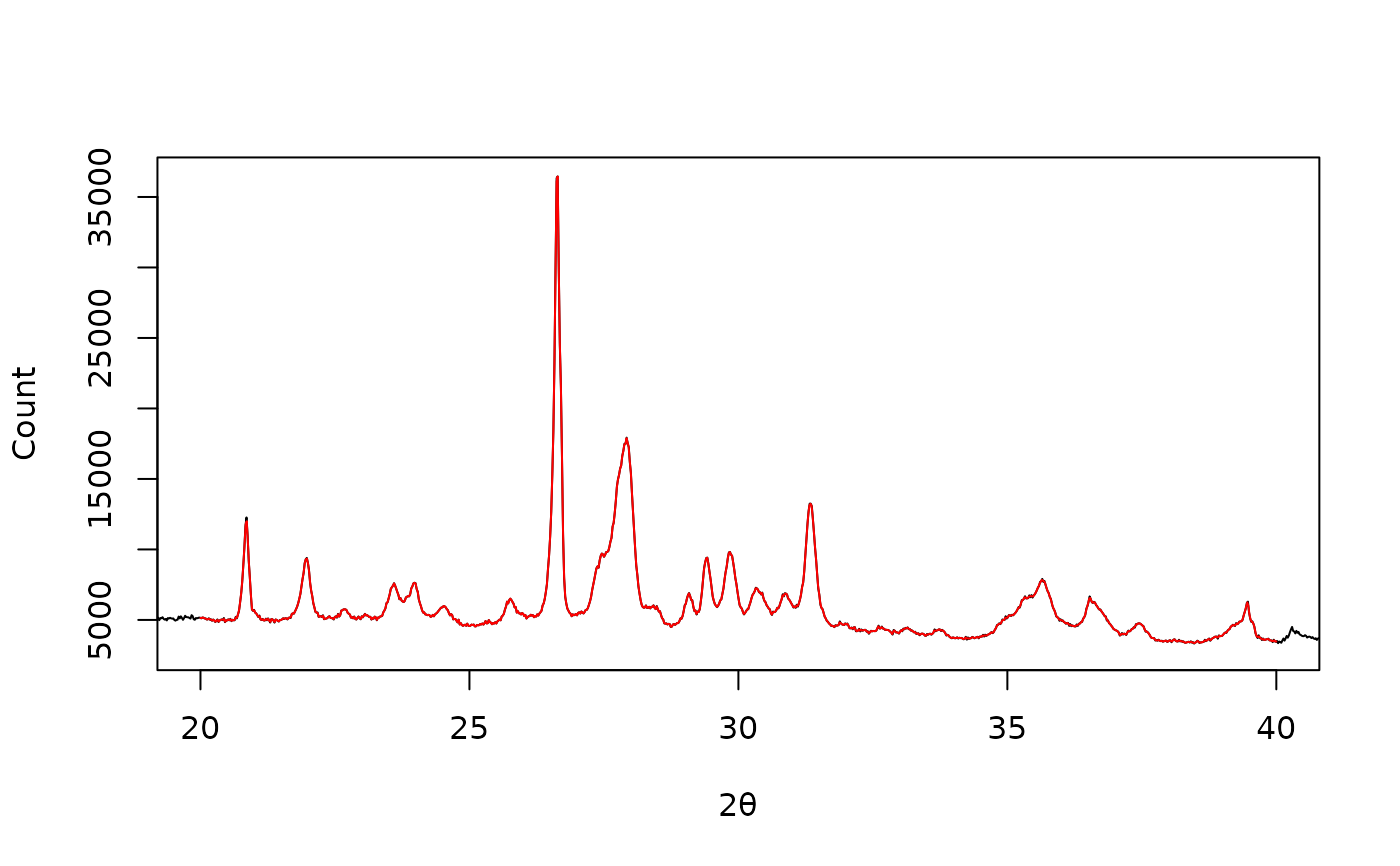Downsample

## Usage

resample_down(x, y, ...)

# S4 method for numeric,numeric
resample_down(x, y, by)

# S4 method for ANY,missing
resample_down(x, y, by)

## Arguments

x, y

A numeric vector. If y is missing, an attempt is made to interpret x in a suitable way (see grDevices::xy.coords()).

...

Currently not used.

by

An integer specifying the downsampling factor.

## Value

Returns a list with two components x and y.

Other resampling methods: resample_bin(), resample_interpolate()

N. Frerebeau

## Examples

## X-ray diffraction
data("XRD")

## Plot spectrum
plot(XRD, type = "l", xlab = expression(2*theta), ylab = "Count")## Bin by 3
XRD_bin_mean <- resample_bin(XRD, by = 3, f = mean)
XRD_bin_min <- resample_bin(XRD, by = 3, f = min)

plot(XRD, type = "l", xlim = c(25, 35),
xlab = expression(2*theta), ylab = "Count")
lines(XRD_bin_mean, type = "l", col = "red")
lines(XRD_bin_min, type = "l", col = "green")## Downsample by 10
XRD_down <- resample_down(XRD, by = 10)

plot(XRD, type = "l", xlim = c(20, 40),
xlab = expression(2*theta), ylab = "Count")
lines(XRD_down, type = "l", col = "red")## Linearly interpolate
XRD_approx <- resample_interpolate(XRD, from = 20, to = 40, by = 0.02)

plot(XRD, type = "l", xlim = c(20, 40),
xlab = expression(2*theta), ylab = "Count")
lines(XRD_approx, type = "l", col = "red")